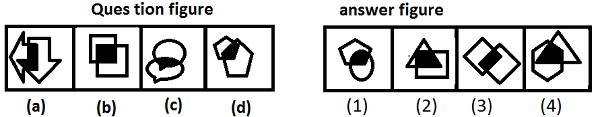# Figure Series - Solved Examples

Q 1 − Which figure must continue series of figure?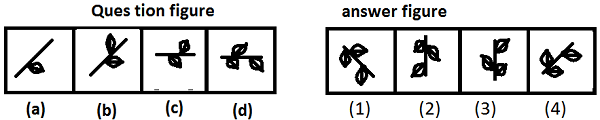Explanation

In each step, one leaf is getting added on either side of line and after each two steps, figure is rotating through an angle of 45° clockwise. During rotation no leaf is added.

Q 2 − Which figure must continue series of figure?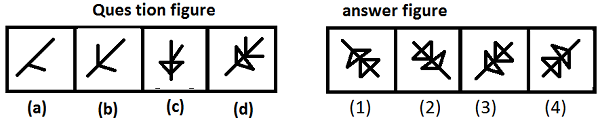Explanation

In each step one line is added to either side of inclined line to make triangular shape and figure is rotating through an angle of 45° anti clockwise.

Q 3 − Which figure must replace question mark?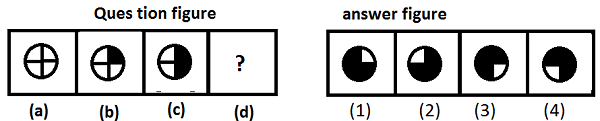Explanation

In each step one quarter of the figure is shaded in clockwise direction.

Q 4 − Which figure must replace question mark?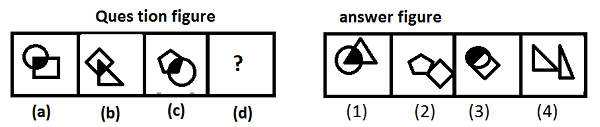Explanation

Each figure has shaded common area.

Q 5 − Which figure is not following the same sequence as followed by figures in question figure?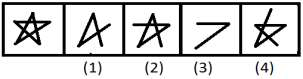Explanation

In each step 2 lines are getting omitted while one line is added in following step.

Q 6 − Which figure is not following the same sequence as followed by figures in question figure?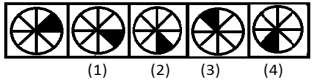Explanation

In each step, one space is shaded in clockwise direction.

Q 7 − Find the answer figure from alternatives which are numbered from 1 to 4 by using the sequence of set of figures given in question figure.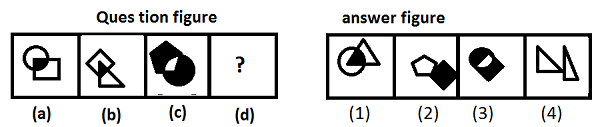Explanation

Like in 1st two figures common area is shaded, in next set of figures common area is not shaded.

Q 8 − Find the answer figure from alternatives which are numbered from 1 to 4 by using the sequence of set of figures given in question figure.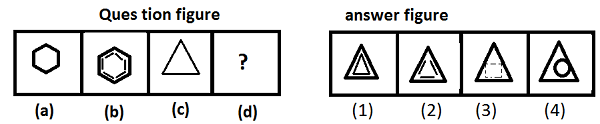Explanation

Parallel lines are added to each side of the figure.

Q 9 − Which figure is different from others?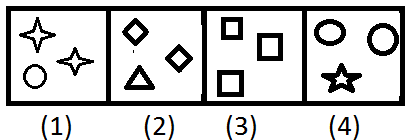Explanation

Each box has three figures of two types, of which two are same while other is different.

Q 10 − Which figure is similar to question figure?